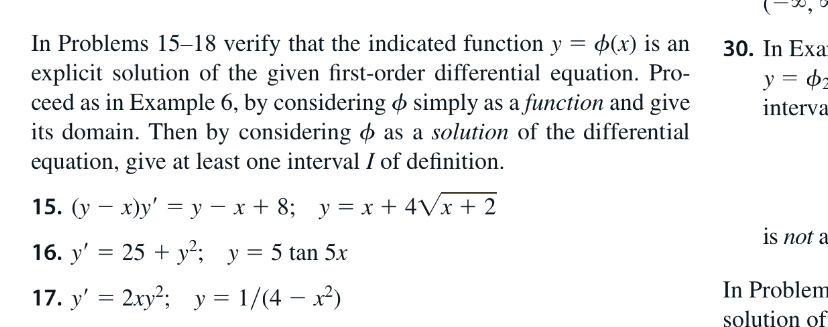# In Problems 15–18 verify that the indicated function y explicit solution of the given first-order differential equation. Pro- ceed as in Example 6, by considering o simply as a function and give its domain. Then by considering o as a solution of the differential equation, give at least one interval I of definition. 30. In Exa у 3 ф interva 15. (у — х)у' %—у — х+ 8; у%3Dх + 4Vx + 2 is not a 16. y' = 25 + y²; y= 5 tan 5x In Problem solution of 17. y' = 2xy²; y = 1/(4 – x) %3D

Question

#17help_outlineImage TranscriptioncloseIn Problems 15–18 verify that the indicated function y explicit solution of the given first-order differential equation. Pro- ceed as in Example 6, by considering o simply as a function and give its domain. Then by considering o as a solution of the differential equation, give at least one interval I of definition. 30. In Exa у 3 ф interva 15. (у — х)у' %—у — х+ 8; у%3Dх + 4Vx + 2 is not a 16. y' = 25 + y²; y= 5 tan 5x In Problem solution of 17. y' = 2xy²; y = 1/(4 – x) %3D fullscreen

## Expert Answer

1 Rating

### Want to see this answer and more?

Experts are waiting 24/7 to provide step-by-step solutions in as fast as 30 minutes!*

*Response times vary by subject and question complexity. Median response time is 34 minutes and may be longer for new subjects.
Tagged in
MathCalculus

### Derivative© 2021 bartleby. All Rights Reserved.